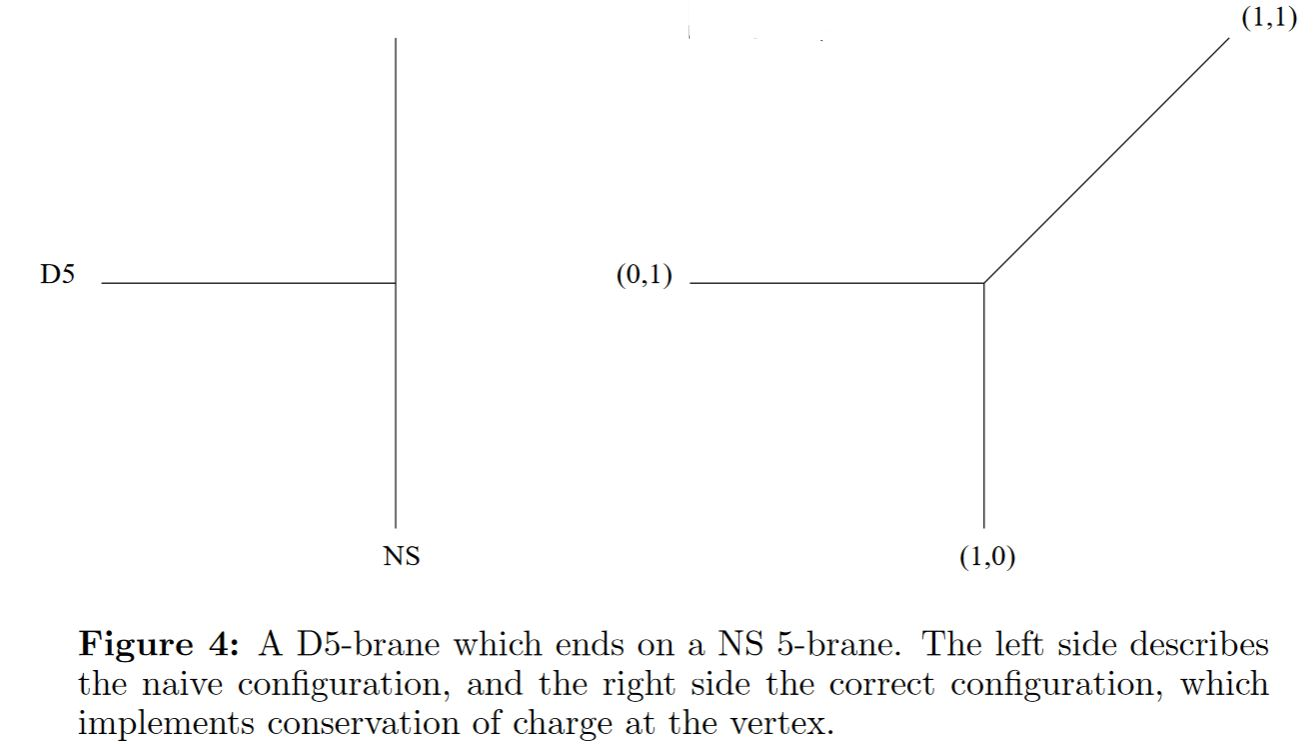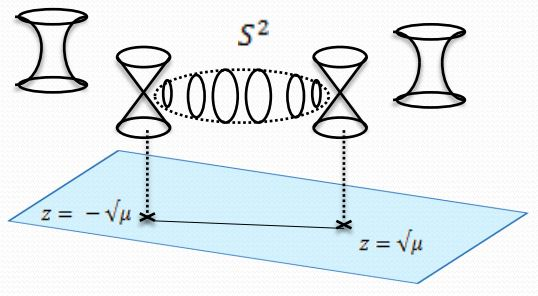# nLab (p,q)5-brane

Contents

## Surveys, textbooks and lecture notes

#### Gravity

gravity, supergravity

## Spin geometry

spin geometry

Dynkin labelsp. orth. groupspin grouppin groupsemi-spin group
SO(2)Spin(2)Pin(2)
B1SO(3)Spin(3)Pin(3)
D2SO(4)Spin(4)Pin(4)
B2SO(5)Spin(5)Pin(5)
D3SO(6)Spin(6)
B3SO(7)Spin(7)
D4SO(8)Spin(8)SO(8)
B4SO(9)Spin(9)
D5SO(10)Spin(10)
B5SO(11)Spin(11)
D6SO(12)Spin(12)
$\vdots$$\vdots$
D8SO(16)Spin(16)SemiSpin(16)
$\vdots$$\vdots$
D16SO(32)Spin(32)SemiSpin(32)

string geometry

# Contents

## Idea

In type IIB string theory there are bound states of D5-branes with NS5-branes. The bound state of $p \in \mathbb{Z}$ D5-branes with $q \in\mathbb{Z}$ NS5-branes is then called a $(p,q)$-fivebrane or similar.

As $p$ and $q$ varies the species of $(p,q)$5-branes form the lattice $\mathbb{Z}^2$ and are naturally acted on by the S-duality group SL(2,Z).

## Properties

### $(p,q)$-Brane webs

Label local coordinate functions $x^a$ on 10d Minkowski spacetime $\mathbb{R}^{9,1}$ by $0123455'6789$ and write $v_a \coloneqq \partial_{x^a}$ for the corresponding vector field

Consider a $(1,0)$5-brane (a D5-brane) along the multivector field $v_0 v_1 v_2 v_3 v_4 v_5$ and a $(0,1)$5-brane (an NS-brane) along $v_0 v_1 v_2 v_3 v_4 v_{5'}$

$a =$$0$$1$$2$$3$$4$$5$$5'$$6$$7$$8$$9$
D5
NS5

Charge conservation implies that at the brane intersection of the two a $(1,1)$5-brane emerges stretched diagonally along $v_5 + v_{g'}$, i.e. along the multivector field $v_0 v_1 v_2 v_3 v_4 (v_5 + v_{5'})$

Aharony-Hanany 97, Sec. 3The worldvolume-quantum field theory at the brane intersection point is a geometric engineering of D=5 N=1 SYM .

The T-dual perspective are D4/NS5-brane webs (Witten 97).

The result of connecting several such brane intersections are called $(p,q)$-5brane webs (Aharony-Hanany-Krol 97).

brane intersections/bound states/wrapped branes/polarized branes

S-duality$\,$bound states:

intersecting$\,$M-branes:

from M-branes to F-branes: superstrings, D-branes and NS5-branes

M-theory on $S^1_A \times S^1_B$-elliptic fibrationKK-compactification on $S^1_A$type IIA string theoryT-dual KK-compactification on $S^1_B$type IIB string theorygeometrize the axio-dilatonF-theory on elliptically fibered-K3 fibrationduality between F-theory and heterotic string theoryheterotic string theory on elliptic fibration
M2-brane wrapping $S_A^1$double dimensional reduction $\mapsto$type IIA superstring$\mapsto$type IIB superstring$\mapsto$$\mapsto$heterotic superstring
M2-brane wrapping $S_B^1$$\mapsto$D2-brane$\mapsto$D1-brane$\mapsto$
M2-brane wrapping $p$ times around $S_A^1$ and $q$ times around $S_B^1$$\mapsto$$p$ strings and $q$ D2-branes$\mapsto$(p,q)-string$\mapsto$
M5-brane wrapping $S_A^1$double dimensional reduction $\mapsto$D4-brane$\mapsto$D5-brane$\mapsto$
M5-brane wrapping $S_B^1$$\mapsto$NS5-brane$\mapsto$NS5-brane$\mapsto$$\mapsto$NS5-brane
M5-brane wrapping $p$ times around $S_A^1$ and $q$ times around $S_B^1$$\mapsto$$p$ D4-brane and $q$ NS5-branes$\mapsto$(p,q)5-brane$\mapsto$
M5-brane wrapping $S_A^1 \times S_B^1$$\mapsto$$\mapsto$D3-brane$\mapsto$
KK-monopole/A-type ADE singularity (degeneration locus of $S^1_A$-circle fibration, Sen limit of $S^1_A \times S^1_B$ elliptic fibration)$\mapsto$D6-brane$\mapsto$D7-branes$\mapsto$A-type nodal curve cycle degeneration locus of elliptic fibration(Sen 97, section 2)SU-gauge enhancement
KK-monopole orientifold/D-type ADE singularity$\mapsto$D6-brane with O6-planes$\mapsto$D7-branes with O7-planes$\mapsto$D-type nodal curve cycle degeneration locus of elliptic fibration(Sen 97, section 3)SO-gauge enhancement
exceptional ADE-singularity$\mapsto$$\mapsto$$\mapsto$exceptional ADE-singularity of elliptic fibration$\mapsto$E6-, E7-, E8-gauge enhancement

(e.g. Johnson 97, Blumenhagen 10)

The original articles are:

The T-dual perspective are D4/NS5-brane webs:

Further intersection with orientifolds:

• Amihay Hanany, Alberto Zaffaroni, Issues on Orientifolds: On the brane construction of gauge theories with $SO(2n)$ global symmetry, JHEP 9907 (1999) 009 (arXiv:hep-th/9903242)

• Hirotaka Hayashi, Sung-Soo Kim, Kimyeong Lee, Masato Taki, Futoshi Yagi, More on 5d descriptions of 6d SCFTs, JHEP10 (2016) 126 (arXiv:1512.08239)

• Gabi Zafrir, Brane webs in the presence of an $O5^-$-plane and 4d class S theories of type D, JHEP07 (2016) 035 (arXiv:1602.00130)

• Taro Kimura, Rui-Dong Zhu, Section 2 and 3 of Web Construction of ABCDEFG and Affine Quiver Gauge Theories (arXiv:1907.02382)

On $(p,q)$-5-branes as defect branes: